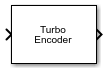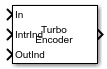# Turbo Encoder

Encode binary data using parallel concatenated encoding scheme

• Library:
• Communications Toolbox / Error Detection and Correction / Convolutional

•## Description

The Turbo Encoder block encodes a binary input signal using a parallel concatenated coding scheme. This coding scheme employs two identical convolutional encoders and one internal interleaver. Each constituent encoder is independently terminated by tail bits. For more information about the constituent encoders, see Parallel Concatenated Convolutional Encoding Scheme.

This icon shows the block with all ports enabled.## Ports

### Input

expand all

Input message, specified as a binary column vector of length L, where L is the length of the uncoded input message.

Data Types: `double` | `int8` | `fi(data,0,1)`

Interleaver indices, specified as a column vector of integers. The vector must be of length L, where L is the length of the binary input message. Each element of the vector must be an integer in the range [1, L] and must be unique. The interleaver indices define the mapping used to permute the input bits at the encoder.

#### Dependencies

To enable this port, set the Source of interleaver indices property to ```Input port```.

Data Types: `double`

Output indices for the bit ordering and puncturing used on the fully encoded data, specified as a column vector of integers. Element values in the vector must be relative to the fully encoded data for the coding scheme, including the tail bits for all streams.

Tunable: Yes

#### Dependencies

To enable this port, set the Source of output indices property to `Input port`.

Data Types: `double`

### Output

expand all

Parallel concatenated codeword, returned as a binary column vector of length M, where M is the number of bits in the parallel concatenated codeword. This output inherits its data type from the `In` input.

Data Types: `double` | `int8` | `fi(data,0,1)`

## Parameters

expand all

Specify the trellis as a MATLAB® structure that contains the trellis description for a rate KN constituent convolutional code. K is the number of input bit streams, and N is the number of output bit streams.

Note

K must be 1 for the turbo coder. For more information, see Coding Rate.

You can either use the `poly2trellis` function to create the trellis structure or create it manually. For more about this structure, see Trellis Description of a Convolutional Code and the `istrellis` function.

The trellis structure contains these fields.

Number of symbols input to the encoder, specified as an integer equal to 2K, where K is the number of input bit streams.

Data Types: `double`

Number of symbols output from the encoder, specified as an integer equal to 2N, where N is the number of output bit streams.

Data Types: `double`

Number of states in the encoder, specified as a power of 2.

Data Types: `double`

Next states for all combinations of current states and current inputs, specified as a matrix of integers. The matrix size must be `numStates`-by-2K.

Data Types: `double`

Outputs for all combinations of current states and current inputs, specified as a matrix of octal numbers. The matrix size must be `numStates`-by-2K.

Data Types: `double`

Specify the source of the interleaver indices as `Property` or ```Input port```.

• When you set this parameter to `Property`, the block uses the Interleaver indices parameter to specify the interleaver indices.

• When you set this parameter to ```Input port```, the block uses the `IntrInd` input port to specify the interleaver indices.

Specify the interleaver indices as a column vector of integers. The vector must be of length L, where L is the length of the binary input message. Each element of the vector must be an integer in the range [1, L] and must be unique. The interleaver indices define the mapping used to permute the input bits at the encoder.

#### Dependencies

To enable this parameter, set the Source of interleaver indices parameter to `Property`.

Specify the source of the output indices as `Auto`, `Property`, or `Input port`.

• When you set this parameter to `Auto`, the block computes output indices that puncture the second systematic stream and include all tail bits.

• When you set this parameter to `Property`, the block uses the output indices that you specify for the Output indices parameter.

• When you set this parameter to ```Input port```, the block uses the OutInd input port to specify the output indices. The vector length and values for the output indices and coded output signal can change with each execution of the block.

Specify the output indices for the bit ordering and puncturing used on the fully encoded data as a column vector of integers. The number of bits output from the encoder is equal to the length of this parameter. The maximum length must not exceed the fully encoded length of (L+mLen) × N × 2), where L is the input vector length, mLen is the memory length, and N is the number of constituent coder encoded streams.

#### Dependencies

To enable this parameter, set the Source of output indices parameter to `Property`.

Type of simulation to run, specified as ```Interpreted execution``` or `Code generation`.

• `Interpreted execution` –– Simulate the model by using the MATLAB interpreter. This option requires less startup time than the `Code generation` method, but the speed of subsequent simulations is slower. In this mode, you can debug the source code of the block.

• `Code generation` –– Simulate the model by using generated C code. The first time you run a simulation, Simulink® generates C code for the block. The C code is reused for subsequent simulations unless the model changes. This option requires additional startup time, but the speed of the subsequent simulations is faster than `Interpreted execution`.

## Block Characteristics

 Data Types `Boolean` | `double` | `fixed point[a]` | `integer` | `single` Multidimensional Signals `no` Variable-Size Signals `yes` [a] ufix(1) only.

expand all

 Berrou, C., A. Glavieux, and P. Thitimajshima. “Near Shannon Limit Error-Correcting Coding and Decoding: Turbo-Codes.” Proceedings of ICC 93 - IEEE International Conference on Communications, Geneva, Switzerland, May 1993, 1064–70. https://doi.org/10.1109/icc.1993.397441.

 Benedetto, S., G. Montorsi, D. Divsalar, and F. Pollara. "A Soft-Input Soft-Output Maximum A Posterior (MAP) Module to Decode Parallel and Serial Concatenated Codes." Jet Propulsion Lab TDA Progress Report, 42–127, (November 1996).

 Schlegel, Christian, and Lance Perez. Trellis and Turbo Coding. IEEE Press Series on Digital & Mobile Communication. Piscataway, NJ ; Hoboken, NJ: IEEE Press ; Wiley-Interscience, 2004.

 3GPP TS 36.212. "Multiplexing and channel coding." 3rd Generation Partnership Project; Technical Specification Group Radio Access Network; Evolved Universal Terrestrial Radio Access (E-UTRA). https://www.3gpp.org.